# Relational Operators in Oracle

## Relational Operators in Oracle with Examples

In this article, I am going to discuss Relational Operators in Oracle with Examples. Please read our previous article, where we discussed Assignment Operators in Oracle with Examples.

##### What are Comparison/Relational Operators in Oracle?

As the name suggests the Relational/Comparison Operators in Oracle are used to compare two values i.e. these operators are used for comparing one expression with another expression. The relational operators determine whether the two values are equal or a value is greater than the other, or less than the other. The result of a comparison can be TRUE, FALSE, or NULL (When one or both the expressions contain NULL values).

##### Types of Relational Operators in Oracle

The different types of relational operators that are available in Oracle are as follows:

1. Equal (=) Operator
2. Not Equal (!= or <>) Operator
3. Greater Than (>) Operator
4. Less Than (<) Operator
5. Greater Than or Equal To (>=) Operator
6. Less Than or Equal To (<=) Operator
##### Understanding the Relational Operators in Oracle with Examples:

Let us understand how to use the relational operators with some examples. We are going to use the following Employee table to understand these operators.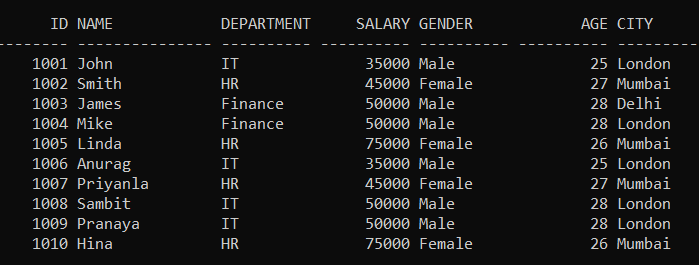Please execute the below SQL query to drop the existing Employee table and Create a new Employee table with the required sample data. We also set the linesize to get the output in the above format.

```SET linesize 300;

DROP Table Employee;

CREATE TABLE Employee (
Id INT PRIMARY KEY,
Name VARCHAR(15),
Department VARCHAR(10),
Salary NUMBER(8, 2),
Gender VARCHAR(10),
Age INT,
City VARCHAR(10)
);

INSERT INTO Employee (Id, Name, Department, Salary, Gender, Age, City) VALUES (1001, 'John', 'IT', 35000, 'Male', 25, 'London');
INSERT INTO Employee (Id, Name, Department, Salary, Gender, Age, City) VALUES (1002, 'Smith', 'HR', 45000, 'Female', 27, 'Mumbai');
INSERT INTO Employee (Id, Name, Department, Salary, Gender, Age, City) VALUES (1003, 'James', 'Finance', 50000, 'Male', 28, 'Delhi');
INSERT INTO Employee (Id, Name, Department, Salary, Gender, Age, City) VALUES (1004, 'Mike', 'Finance', 50000, 'Male', 28, 'London');
INSERT INTO Employee (Id, Name, Department, Salary, Gender, Age, City) VALUES (1005, 'Linda', 'HR', 75000, 'Female', 26, 'Mumbai');
INSERT INTO Employee (Id, Name, Department, Salary, Gender, Age, City) VALUES (1006, 'Anurag', 'IT', 35000, 'Male', 25, 'London');
INSERT INTO Employee (Id, Name, Department, Salary, Gender, Age, City) VALUES (1007, 'Priyanla', 'HR', 45000, 'Female', 27, 'Mumbai');
INSERT INTO Employee (Id, Name, Department, Salary, Gender, Age, City) VALUES (1008, 'Sambit', 'IT', 50000, 'Male', 28, 'London');
INSERT INTO Employee (Id, Name, Department, Salary, Gender, Age, City) VALUES (1009, 'Pranaya', 'IT', 50000, 'Male', 28, 'London');
INSERT INTO Employee (Id, Name, Department, Salary, Gender, Age, City) VALUES (1010, 'Hina', 'HR', 75000, 'Female', 26, 'Mumbai');
```
##### Equal (=) Relational Operator in Oracle

The Equal (=) Operator in Oracle is used to check whether the two expressions are equal or not. If both the expressions are equal then the condition becomes true and will return the matched rows. For example, the following SQL Query will return all the records from the Employee table where the Gender is Male. Here the expression is Gender = ‘Male’ and it will check all the Gender column values in the Employee table and those values which are Matched with the value Male will be returned.

SELECT * FROM Employee WHERE Gender = ‘Male’;

Once you execute the above SELECT SQL Statement, you will get the following result set which includes only the employees whose gender is Male. In our Employee table, 6 employees having gender Male and those 6 employees will return as part of the result set.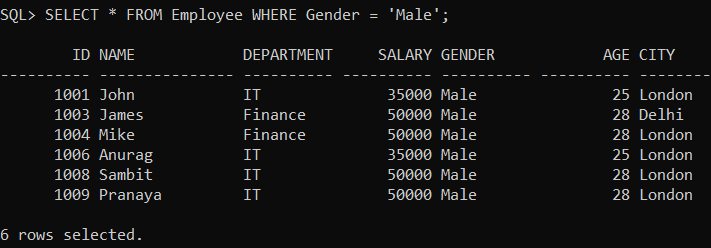##### Not Equal (!=) Relational Operator in Oracle

The Not Equal (!=) Operator in Oracle is just the opposite of the equal operator. That means this operator is used to check whether the two expressions are equal or not. If both the expressions are not equal then the condition becomes true and will return the not-matched records. For example, the below SQL Statement will return all records from the Employee table except the employees whose gender is Male.

SELECT * FROM Employee WHERE Gender != ‘Male’;

Once you execute the above Select SQL Statement, then you will get the following result set which includes only the Female employees.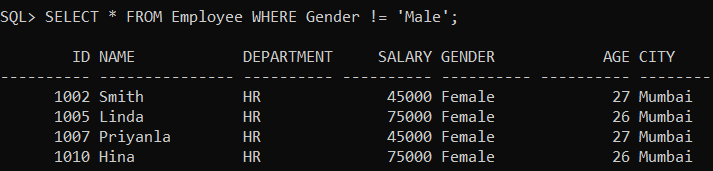##### Not Equal (<>) Relational Operator in Oracle

The Not Equal (<>) Operator in Oracle is the same as the Not Equal (!=) operator. That means it is also used to check whether the two expressions are equal or not. If both the expressions are not equal then the condition becomes true and will return the not-matched records. Let us take the same example as the previous one. In the below example, it will return all records from the Employee table except the employees whose gender is Male.

SELECT * FROM Employee WHERE Gender <> ‘Male’;

The above SQL Query will give the same output as the previous example as shown in the below image.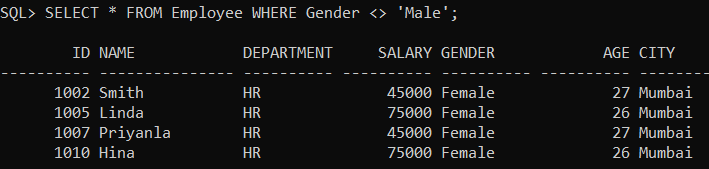##### Greater Than (>) Relational Operator in Oracle

The Greater Than (>) Operator in Oracle is used to check whether the left-hand expression value is higher than the right-hand expression value. If the left-hand expression value is higher than the right-hand expression value then the condition becomes true and it will return the matched records. For example, the below SQL query will return all the records from the Employee table where the employee salary is greater than 45000.

SELECT * FROM Employee WHERE Salary > 45000;

Once you execute the above Select SQL query, you will get the following result set which includes all the employees whose salary is greater than 45000.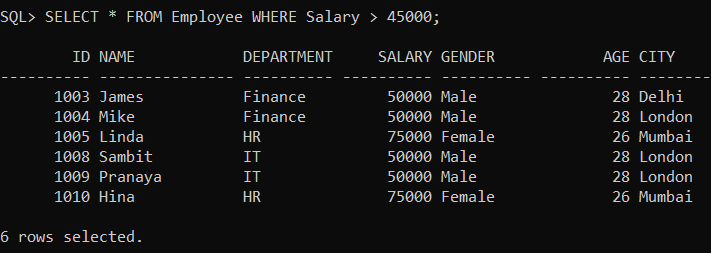Note: This operator is used for the Greater than test. For example, a>b checks the operand ‘a’ is greater than ‘b’ or not.

##### Less Than (<) Relational Operator in Oracle

The Less Than (>) Operator in Oracle is used to check whether the left-hand expression value is lower than the right-hand expression value. If the left-hand expression value is lower than the right-hand expression value then the condition becomes true and will return the matched records. For example, the below SQL query will return all records from the Employee table where the employee salary is less than 50000.

SELECT * FROM Employee WHERE Salary < 50000;

Once you execute the above SELECT SQL Statement, then you will get the following result set which includes all the employees whose salary is less than 50000.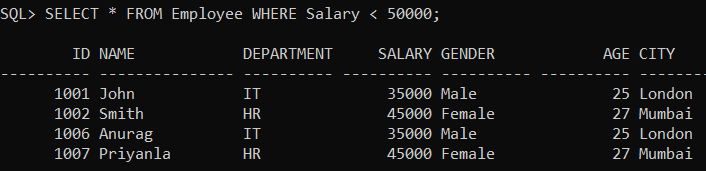Note: This operator is used for less than test. Example a<b checks that operand ‘a’ is less than ‘b’ or not.

##### Greater Than or Equal To (>=) Operator in Oracle

The Greater than or Equal To (>=) Operator in Oracle is used to check whether the left-hand expression value is higher than or equals to the right-hand expression value or not. If the left-hand expression value is higher than or equals to the right-hand expression value then the condition becomes true and will return all the matched records. For example, the following SQL Query will return all the records from the Employee table where the Salary is greater than or equal to 50000.

SELECT * FROM Employee WHERE Salary >= 50000;

When you execute the above Select query, then you will get the following result set which includes all the employees whose salary is greater than or equals 50000.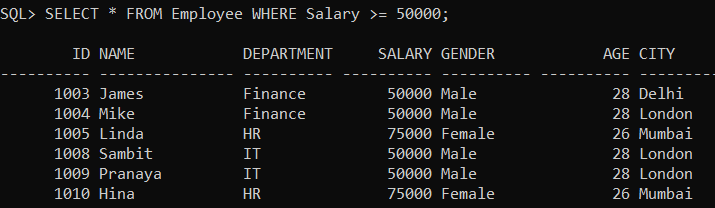Note: This operator is used to check the Greater than or equal test. For example, a>=b checks the operand ‘a’ is greater than operand ‘b’ or operand ‘a’ is equals to the operand ‘b’.

##### Less Than or Equal To (<=) Operator in Oracle

The Less than or Equal To (<=) Operator in Oracle is used to check whether the left-hand expression value is lower than or equal to the right-hand expression value or not. If the left-hand expression value is lower than or equals to the right-hand expression value then the condition becomes true and it will return all the matching records. For example, the following SQL Query will return all the records from the Employee table where the Salary is less than or equal to 50000.

SELECT * FROM Employee WHERE Salary <= 50000;

When you execute the above SQL Statement, then you will get the following result set which includes all the employees whose salary is less than or equals 50000.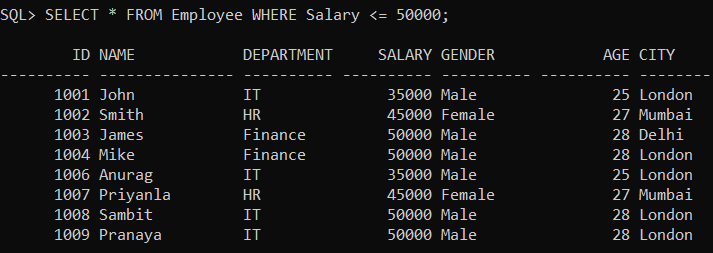Note: This operator is used for Less than or Equal to test. For example, a<=b, here checks whether operand ‘a’ is less than or equals to operand ‘b’. If a<b then the condition is true and if a=b then also condition is true but if a>b then the condition is false.

In the next article, I am going to discuss Logical AND OR & NOT Operators in Oracle with Examples. Here, in this article, I try to explain Relational Operator in Oracle with Examples and I hope you enjoy this Relational Operators in Oracle with Examples article.